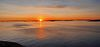# Patrik Jansson

More### Patrik Jansson

Professor of Computer Science

+46317725415

# Domain-Specific Languages of Mathematics

The BSc level course Domain-Specific Languages of Mathematics (course code DAT326 at Chalmers and DIT982 at UGOT) presents classical mathematical topics from a computing science perspective: giving specifications of the concepts introduced, paying attention to syntax and types, and ultimately constructing DSLs of some mathematical areas mentioned below.
Lectures:
• Introduction: Haskell, complex numbers, syntax, semantics, evaluation, approximation
• Basic concepts of analysis: sequences, limits, convergence, ...
• Types and mathematics: logic, quantifiers, proofs and programs, Curry-Howard, ...
• Type classes, derivatives, differentiation, calculational proofs
• Domain-Specific Languages and algebraic structures, algebras, homomorphisms
• Polynomials, series, power series
• Power series and differential equations, exp, sin, log, Taylor series, ...
• Linear algebra: vectors, matrices, functions, bases, dynamical systems as matrices and graphs
• Laplace transform: exp, powers series cont., solving PDEs with Laplace
The course has been running yearly from  2016 and from 2022 there is a course book published by College Publications
Share